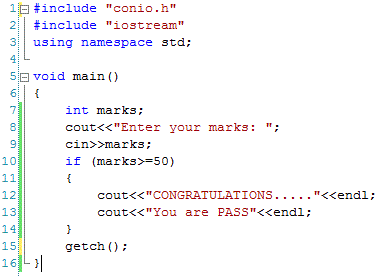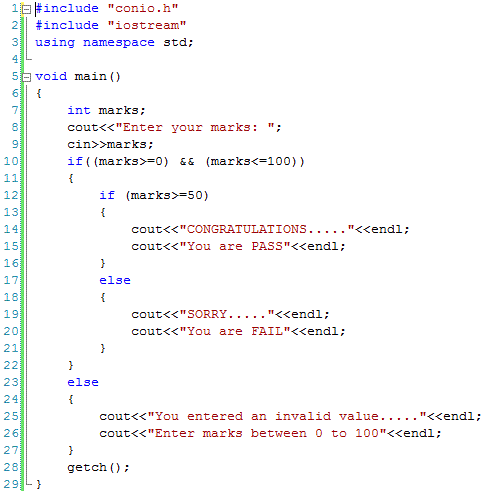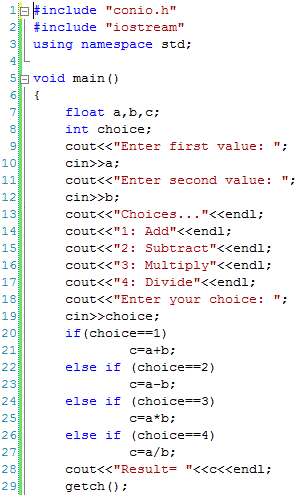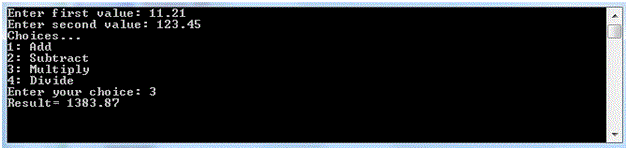# Decision making in C Plus Plus

## Decision making in C++

For this purpose the following decision making statements are provided in C++. if statement, switch statement and conditional operator.

Like most other computer languages, statements in C++ are executed one after another from top to bottom. But sometimes we may need that some statement(s) should be executed before checking a certain condition or we can say that a decision is required to be made that whether a particular statement or the block of statements should be executed or not. For this purpose the following decision making statements are provided in C++.

• if statement
• switch statement
• conditional operator

if statement

The simplest form of if statement is as below:

if (condition)
statement1;
statement2;
statement3;

In the above statement, condition is an expression using relational or logical operators. The result of a condition may be either true or false. If the condition is true then statement1 will be executed otherwise skipped. Kindly note that statement2 and statement3 are not within if statement. These statements will be executed whether condition is true or false. If we want that both statement2 and statement3 should also be skipped if condition is false then we need to write the if statement as below.

if (condition)
{
statement1;
statement2;
statement3;
}

Now statement1, statement2 and statement3 can only be executed if condition is true otherwise all of these statements will be skipped. The following example shows the use of if statement. It will get marks from the user. If marks are greater than or equal to 50 then it will display a congratulations message.

Example:The output of this program is as below.if-else statement

The problem with the above example is that if marks are greater than or equal to 50 then it executes the body of if statement otherwise nothing happens. A more appropriate statement could be to display a message that you are FAIL if marks are not greater than 50. For this purpose we can use an if-else statement as below.

if (condition)
{
statement1;
statement2;
statement3;
}
else
{
statement1;
statement2;
}
In this case, all the statements within if block are executed if the condition is true otherwise the statement written in the else block are executed. It is important to note that either if block or else block is executed depending upon the result of condition. It is not possible that both if and else blocks are executed.

Example:The output of the above program is as below if marks are less than 50.Nested if statements

If we write an if statement within another if statement then this is called nested if statements. The inner if may be in the if block or in the else block or in the both blocks as explained below. It is important to note that it is not required to use the else part of if statement while nesting but may be used if required.

if (condition1)
{
if(condition2)
{
Statement1;
Statement2;
———-;
———-;
}
else
{
Statement3;
Statement4;
———-;
———-;
}
}
else
{
if(condition3)
{
Statement5;
Statement6;
———-;
———-;
}
else
{
Statement7;
Statement8;
———-;
———-;
}
}

The execution of this example is explained as below.

 If … If … Block to be executed condition1 is true condition2 is true Statement1;Statement2; ———-; ———-; condition1 is true condition2 is false Statement3;Statement4; ———-; ———-; condition1 is false condition2 is true Statement5;Statement6; ———-; ———-; condition1 is false condition2 is false Statement7;Statement8; ———-; ———-;

The last code example can be modified as below to demonstrate the use of nested if statements. In the following example, user is required to enter an integer between 0-100. If a value is entered between this range then the if block of outer if is executed where the value is again checked that whether to display ‘PASS’ or ‘FAIL’ message. If the given value is not within the expected range then the else block of the outer if is executed to display an appropriate error message.if-else-if statement

This is another form of if statement. If we need to check a number of conditions and want to execute one of the related block then we can use this kind of statement as illustrated below.

if (condition1)
{
Statement1;
Statement2;
———-;
———-;
}
else if (condition2)
{
Statement3;
Statement4;
———-;
———-;
}
else if (condition3)
{
Statement5;
Statement6;
———-;
———-;
}

This kind of if is illustrated in the example below. In the following example, two integer values are accepted from user then a list of choices is displayed. User selects one of the choices and then appropriate result is calculated.The output of the above code is as below.switch statement

switch statement is an alternative to the last if-else-if statement. If we want to execute one of the blocks depending on the various conditions then switch statement can be used as shown below.

switch (variable/expression)
{
case 1:
———-;
———-;
break;
case 2:
———-;
———-;
break;
case 3:
———-;
———-;
break;
.
.
.
default:
———-;
———-;
}

In the above switch statement, same variable or expression is compared with different values. If the value is 1 then first block will be executed. If the value is 2 then second block is executed and so on. If the value of variable/expression does not match with any case value then the default block is executed. It is important to note the break statement at the end of every case block. If the break statement is not used in a case block then the next case block will be executed until a break statement is found or the switch statement ends.

We can replace the previous code example with switch statement as follows.

#include “conio.h”
#include “iostream”
using namespace std;

void main()
{
float a,b,c;
int choice;
cout<<“Enter first value: “; cin>>a;
cout<<“Enter second value: “; cin>>b;
cout<<“Choices…”<<endl;
cout<<“2: Subtract”<<endl;
cout<<“3: Multiply”<<endl;
cout<<“4: Divide”<<endl;
cin>>choice;
switch (choice)
{
case 1:
c=a+b;
break;
case 2:
c=a-b;
break;
case 3:
c=a*b;
break;
case 4:
c=a/b;
break;
}
cout<<“Result= “<<c<<endl;
getch();
}

The output of the above code is as below.Conditional Operator

Conditional operator is a ternary operator and works like an if-else statement. If we have an if-else statement as below.

if (condition)
statement1;
else
statement2;

This statement can be converted into conditional operator as below.

(condition):statement1?statement2;

The following example illustrates the use of conditional operator.

(a>b)?cout<<”A is greater than B”: ”A is not greater than B”;